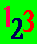The ABC Study Guide, University education in plain English alphabetically indexed. Click here to go to the main index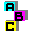# ABC Algebra

Words to help you think about something you do not know. How to be a maths detective like Al'Khwarizmi

Algebra is a more general form of arithmetic that uses symbols (often letters) in place of (some) numbers. The symbols can represent any number, so the results are more general.

Here is an example of arithmetic generalised to algebra:

5 + 3 is the same as 3 + 5. Both equal eight.

We can see that if we alternate the order of the numbers in additions like this, we will not change the answer. Algebra lets us say this general rule symbolically:

(a + b) = (b + a)

Which means that any number (a) added to any other number (b) will have the same sum as the other number (b) added to the original number (a).

Algebra as detection

Algebra is the detective department of mathematics. With algebra we use symbols to mark the parts of the situation we do not know and then see if we can find them out by treating things we do know as the clues. The symbol we use just means "something we do not know". So 2x = y means "two of something we do not know that we will call x equals one of another thing that we do not know that we will call y". Like detectives drawing the possibilities on a flip-chart, we can make a diagram of the possible solutions in the form of a graph. We solve the problem when we find out what x or y is. If we find out one, it tells us the other.

Thought forms like algebra

Using symbols for unknown quantities is a very useful technique. For example, it is used in computing to find files or words or numbers where only part of the name is known. Here the parts we know are called literal characters and the ones we do not know are called wildcards or metacharacters. A combination of literals and wildcards is called a regular expression.

An example of a wildcard is the asterisk (*). If you search for *.exe on a computer you will get a list of all the files with the extension exe, whatever the first part of the name.

Analysis

Analysis in mathematics can refer to

A mathematical function is something that varies in a way dependent on some other item or items.

"x is a function of y" means you should be able to work out x if you know what y is (or y if you know what x is) and what the equation is that relates them

"x is a function of y and z" means you should be able to work out x if you know what y and z are and what the equation is that relates them. "x = 2yz" is an equation relating x, y and z.

Equation

In mathematics, an equation is a statement that two amounts or values are equal. Two very simple equations are:

x = y
y = 10
Equations can have solutions because you can sometimes work out from two of them what the value of an unknown quantity is. For example, from the two equations above, it is possible to work out that x = 10.

Expression
An expression usually refers to an
algebraic expression, which is a combination of symbols which stands for numbers and for operations with them.

2a + 3b is an algebraic expression. Because it has two terms (2a and 3b) it is called a binomial expression.

Variable - Something that changes

We use variables in algebra, in statistics, and in experiments.

Algebraic variables

In arithmetic we use definite numbers, like 1, 236, 0.5, where the quantity referred to does not vary.

In algebra we also use general expressions, like a, b, x, where the symbol represents a number that varies.

In algebra, a variable is a symbol used to represent any number. For example, in this equation:

2(x+y) = 2x + 2y

x and y are variables (general expressions) that represent any number, whereas the definite number 2 is called a constant because it stays the same.

Statistical variables
In Statistics, a variable is data that varies numerically. Examples are a person's age, height or income.

Statistical variables are contrasted with attributes, which are qualitative (non-numerical) descriptions like a person's gender or class.

Experimental variables
In an experimental context, a variable means anything that is free to vary. The experiment will investigate the relationship between the independent variable, which is the one the experimenter alters, and the dependent variable, which is the one affected by the alteration.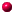Study links outside this site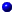Andrew Roberts' web Study GuidePicture introduction to this siteTop of Page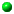Take a Break - Read a Poem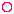Click coloured words to go where you want

Andrew Roberts likes to hear from users:
To contact him, please use the Communication Form

Maths index

*****************

Algebra index

